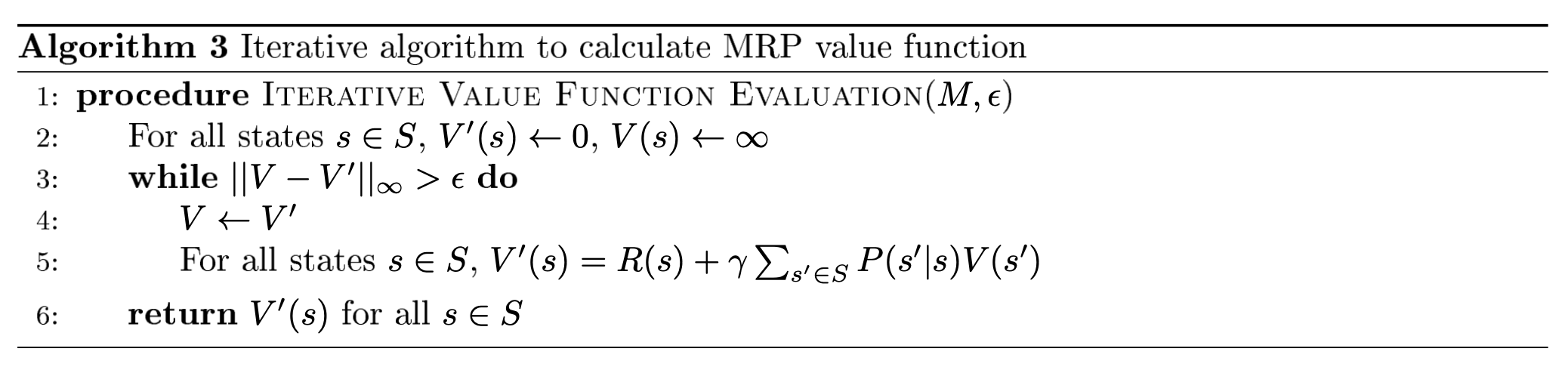N1H111SM's Miniverse

# RL(1) - MP, MRP, MDP

2019/07/10 Share# Markov Reward Process

• $S$：代表环境或者世界的一个有限状态集
• $P$：一个状态转移模型，声明了$P(s_{t+1}=s’|s_t=s)$
• $R$：一个奖励函数，声明了在某一状态下agent收到的奖励，$R(s_t=s)=E[r_t|s_t=s]$
• $\gamma$：对于未来奖励进行加权的折扣因子，$\gamma \in [0,1]$

## Parameter Explanation

### Return

Return $G_t$ 的中文译名我没有关注，在数学上的含义就是从某个时间步$t$开始沿着某个状态$s$的序列获得的奖励之和：

## Computing State Value Function for MRP

### Via dynamic programming

Dynamic Programming with tolerence $\epsilon$：• 定义Bellman Operator，证明这个算子是strict contraction的。
• 根据引理得知，strict contraction的算子只有一个不动点，而$BV=R+\gamma PV=V$，则这个不动点就是真实的$V$。
• 利用三角不等式和Bellman Operator 的性质得到，严格证明式为以下：

# Markov Decision Process

• $S$：代表环境或者世界的一个有限状态集，$s\in S$
• $A$：代表agent能够执行的有限动作集，$a\in A$
• $P$：一个状态转移模型。和MRP不同的是，MDP中的状态转移模型是一个同时关于当前状态$s$和当前行动$a$的函数，声明了$P(s_{t+1}=s’|s_t=s,a_t=a)$
• $R$：一个奖励函数。同样的奖励函数也接受了动作$a$作为其中一个变量，声明了在某一状态下采取某一动作agent收到的奖励，$R(s_t=s,a_t=a)=E[r_t|s_t=s,a_t=a]$ 。需要注意的是这仅仅是这门课程中的表示方法，仔细想一下其实$R$是一个仅关于状态的函数也是正确的。
• $\gamma$：对于未来奖励进行加权的折扣因子，$\gamma \in [0,1]$

# MDP Control

MDP Control目的在于找到一个最优的策略$\pi^*$使得在该策略下的状态价值函数最大化。

## Value Iteration

Bellman Operator也被称作Bellman Backup Operator。算子（Operator）就是作用在一个函数上将函数再进行一次映射。Bellman Operator作用在这里的value function上同时返回一个新的映射到所有状态的函数。

Value Iteration的算法中的迭代部分公式为：

Policy Evaluation相当于计算$B^\pi$的不动点，只需要不断地进行Bellman Operator的变换直到$V$不再变化即可。

## VI v.s. PI

VI算法依次计算Horizon为$k$的最优值，PI则被用于计算一个策略的Infinite Horizon值，并用于选择一个更好的策略，和未来即将介绍的RL重要算法Policy Gradient有非常紧密的联系。简单来说，PI算法首先随机初始化一个策略，通过evaluate这个策略得到关于这个策略的价值函数，然后再不断优化这个策略（取得更大的价值函数值）达到拟合；而VI则是将价值函数初始化为0向量，每一次迭代计算当前价值函数的Bellman Optimality Operator，由于该算子是一个压缩算子，因此具有唯一的不动点，并且该不动点是最优点，最后通过价值函数来取得最优的策略。